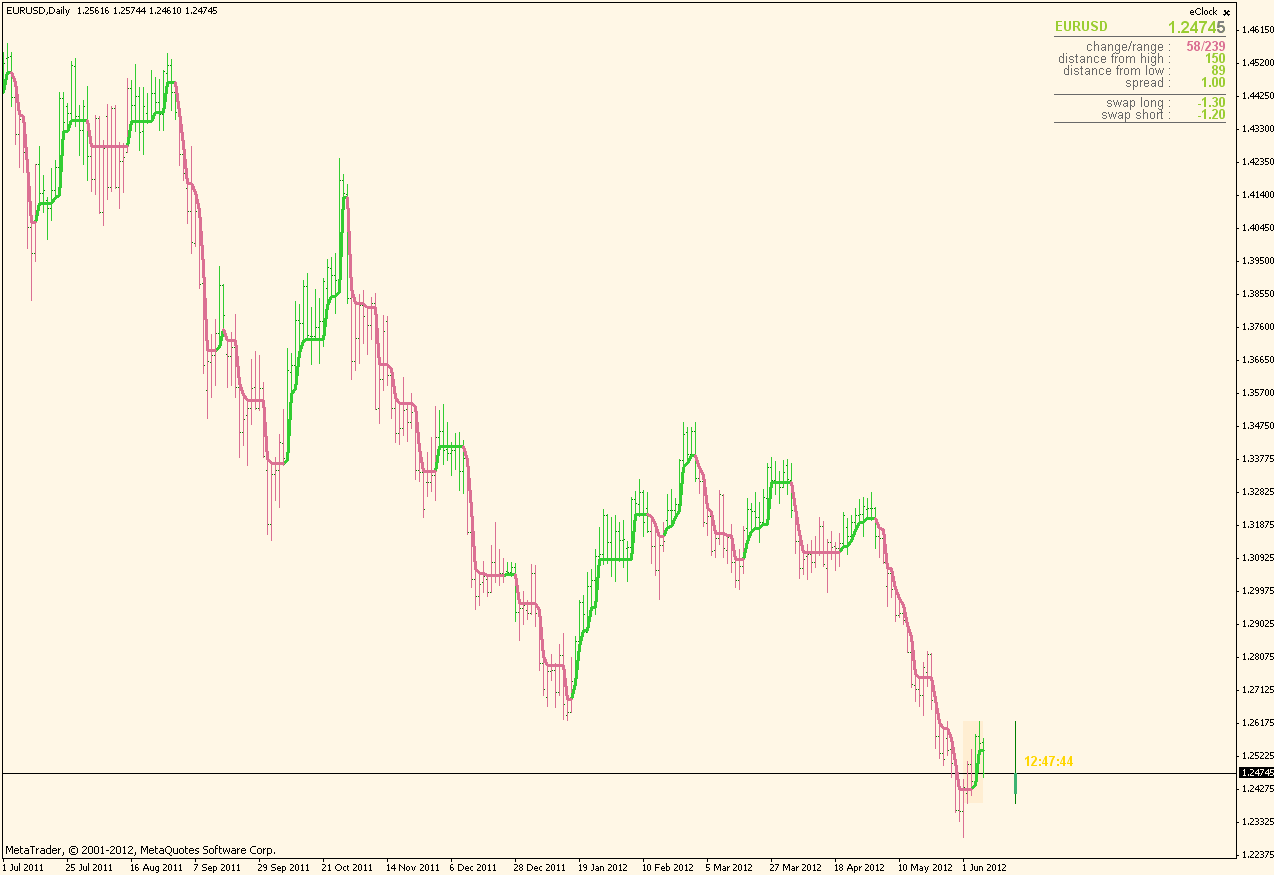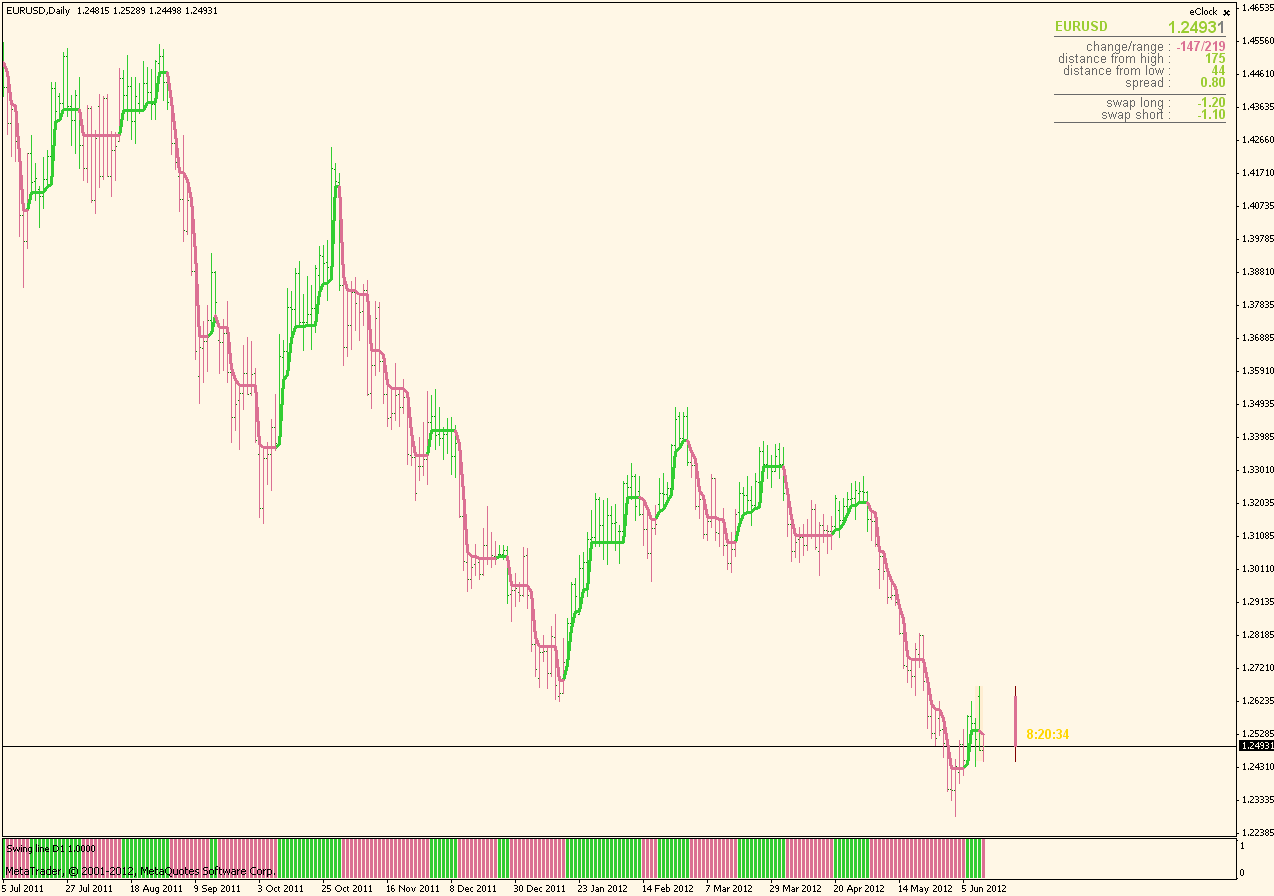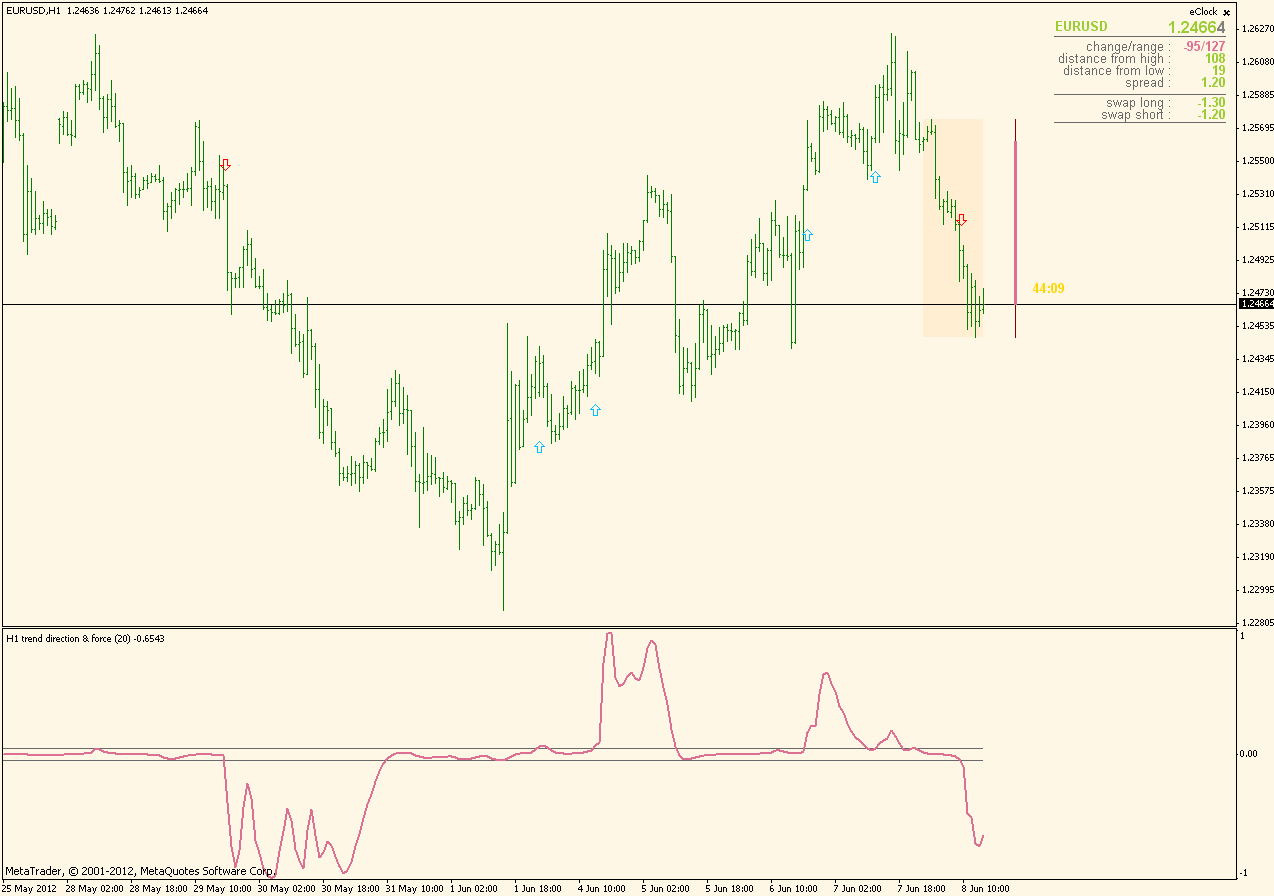Elite indicators :) - page 480151615

...

Ray

That indicator can not be made faster and to have the same values calculated.

The problem is the following : it takes all the symbols that you have allowed in your symbols list and calculates values for those first and then combines them in a "composite value". So it is calculating a lot of symbols and that is the main problem of that indicator being slow. Also, since the number of symbols calculated is nor fixed (it will be different from user to user) I can not make the centered tma calculate faster either, since I can not create a 2 dimensional array that will fit for all needs and cases (metatrader does not allow a variable length for the second dimension of an array, so, since I do not know how many symbols there will be, I can not make an array that would hold centered tma values and that way make the slope calculation at least 50% faster)

Sorry89

This one is regarding the swing line : decided to "renew" it a bit, so it is a "all in one" indicator which allows you to choose if you wish to see lines, bars or candles (or all those together) That way one can easily control all the things it is doing from one single place and choose which way is the best to see it on chart easilyI appreciate your all in one indicator. Is it possible to add drawing dot (same as BB MACD) or histogram style?

Thank you very much.531

Thank you for trying. I use the CSS primarily for just 2 pair at a time on a chart so;

1. CSS; Is there any way to control the number of pairs calculated such as the " showOnlySymbolOnChart". Can the chart pairs determine the pairs to calculate, I suppose I could set up a CSS for each spotpair ?? EURUSD could you look to see what could be re-aligned to just calculate the eurusd?? I have a feeling its not that easy.

2. CCFp; Could you put the "showOnlySymbolOnChart" option in it??.

3. CCFp; you already list the pairs could you show there value in order as the CSS does???

4. CCFp; Any idea on how to get it working on the renko charts??? It could be better then the CSS, it doesn't repaint!!

I know I'm a pain but have you really been challenged this week. If you ignore me I'll understand.

thank you

Ray

Ray

That indicator can not be made faster and to have the same values calculated.

The problem is the following : it takes all the symbols that you have allowed in your symbols list and calculates values for those first and then combines them in a "composite value". So it is calculating a lot of symbols and that is the main problem of that indicator being slow. Also, since the number of symbols calculated is nor fixed (it will be different from user to user) I can not make the centered tma calculate faster either, since I can not create a 2 dimensional array that will fit for all needs and cases (metatrader does not allow a variable length for the second dimension of an array, so, since I do not know how many symbols there will be, I can not make an array that would hold centered tma values and that way make the slope calculation at least 50% faster)

Sorry2970
Hercs

DTosc idea

Could the #DTosc be made into a Candle-colouring indicator?

Looking forward to hearing from you.

Best wishes.151615

...

Ray

The problem with CCFp and renko is in the following code :

double ma(string sym, int per, int Mode, int Price, int i)

{

sym = SymbolsPrefix+sym+SymbolsSuffix;

double res = 0; int k = 1;

switch(Period())

{

case 1: res += iMA(sym, 0, per*k, 0, Mode, Price,i); k += 5;

case 5: res += iMA(sym, 0, per*k, 0, Mode, Price,i); k += 3;

case 15: res += iMA(sym, 0, per*k, 0, Mode, Price,i); k += 2;

case 30: res += iMA(sym, 0, per*k, 0, Mode, Price,i); k += 2;

case 60: res += iMA(sym, 0, per*k, 0, Mode, Price,i); k += 4;

case 240: res += iMA(sym, 0, per*k, 0, Mode, Price,i); k += 6;

case 1440: res += iMA(sym, 0, per*k, 0, Mode, Price,i); k += 4;

case 10080: res += iMA(sym, 0, per*k, 0, Mode, Price,i); k += 4;

case 43200: res += iMA(sym, 0, per*k, 0, Mode, Price,i);

}

return(res);

}

It calculates moving averages from multiple time frames (using the "Elders way" of calculating higher time frame average) and uses that as a result. Now, the first problem is :
1. that renko does not have time frame and it does not know where to enter

2. even if we make it enter at some stage (force it to "behave" like some time frame) the nature of renko (it does not depend on time) makes me wonder what results would we actually get

As of the rest : will see how to make it (it is doable)

Thank you for trying. I use the CSS primarily for just 2 pair at a time on a chart so;

1. CSS; Is there any way to control the number of pairs calculated such as the " showOnlySymbolOnChart". Can the chart pairs determine the pairs to calculate, I suppose I could set up a CSS for each spotpair ?? EURUSD could you look to see what could be re-aligned to just calculate the eurusd?? I have a feeling its not that easy.

2. CCFp; Could you put the "showOnlySymbolOnChart" option in it??.

3. CCFp; you already list the pairs could you show there value in order as the CSS does???

4. CCFp; Any idea on how to get it working on the renko charts??? It could be better then the CSS, it doesn't repaint!!

I know I'm a pain but have you really been challenged this week. If you ignore me I'll understand.

thank you

Ray151615

Swing line histo ...

yuhu

Here you goHisto version of the swing line indicator (compared to the "all-in-one" version on the chart)PS: there was an error in the indicator that prevented it from working correctly in mtf mode. It is corrected now

yuhu:

I appreciate your all in one indicator. Is it possible to add drawing dot (same as BB MACD) or histogram style?

Thank you very much.
Files:2970
Hercs

yuhu

Here you goHisto version of the swing line indicator (compared to the "all-in-one" version on the chart)PS: there was an error in the indicator that prevented it from working correctly in mtf mode. It is corrected now

================

Thanks in advance for fixing it.

Best regards.151615

...

ValeoFX

I can download it (tried it right now and downloaded it without any problem)

What error are you getting?

ValeoFX:
================

Thanks in advance for fixing it.

Best regards.2970
Hercs

Strangely enough it downloaded immediately this morning? Maybe my PC was just tired late yesterday?

Success for the day ahead.

Best regards.52

While I was going through my indicator, I found the coloured version of the forex index smoothed indicator. But whenever acrossing the horizontal line only.

So is it possible of having a coloured indication Regardless the 2 lines. I mean color get changed whenever the value is changing positive or negative.

Here you go